# College Chemistry : Lewis, Brønsted-Lowry, and Arrhenius Definitions

## Example Questions

### Example Question #1 : Lewis, Brønsted Lowry, And Arrhenius Definitions

Identify the Bronsted-Lowry acid in the following equation: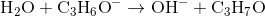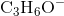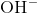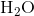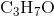Explanation:

Recall that a Bronsted-Lowry acid donates a proton. From the equation, we can see thatbecomes, which it lost an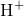, or a proton. Thus, water must be the Bronsted-Lowry acid.

### Example Question #2 : Lewis, Brønsted Lowry, And Arrhenius Definitions

Identify the Lewis Base in the following reaction: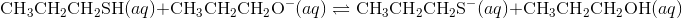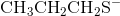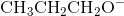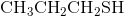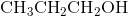Explanation:

Recall that a Lewis base accepts a proton, while a Lewis acid donates a proton. Looking at the equation, we can see thatbecomes. Sinceaccepted a proton, it must be the Lewis base.

### Example Question #3 : Lewis, Brønsted Lowry, And Arrhenius Definitions

Which type of acid is defined by its ability to produce hydrogen ions in aqueous solution?

Bronsted-Lowry acid

Lewis acid

Arrhenius acid

None of these

Arrhenius acid

Explanation:

There are three main definitions for acids and bases: the Lewis acids and bases, Bronsted-Lowry acids and bases, and Arrhenius acids and bases.

A Lewis acid is a species which can accept an electron pair from a donor. An example of a Lewis acid is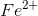; as a cation, it can accept an electron pair. A Lewis base is a species which can donate an electron pair. An example of a Lewis base is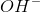; as an anion, it can donate an electron pair.

A Bronsted-Lowry acid is a species which can donate a proton. An example of a Bronsted-Lowry acid is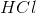, which can donate its hydrogen ion. A Bronsted-Lowry base is a species which can accept a proton from a donor. An example of a Bronsted-Lowry base is, which can accept a hydrogen ion in order to become water.

An Arrhenius acid is a species which releases hydrogen ions into solution. An example of an Arrhenius acid is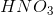. When this compound dissolves in aqueous solution, it produces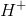and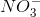. An Arrhenius base is a species which releases hydroxide ions into solution. An example of an Arrhenius base is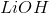. When this compound dissolves in aqueous solution, it produces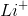and.

### Example Question #4 : Lewis, Brønsted Lowry, And Arrhenius Definitions

What is the pH of a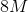solution?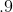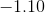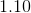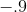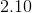Explanation:

PH is defined as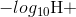, where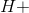is hydronium concentration). In order to do this we simply take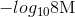: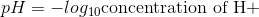=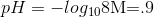### Example Question #5 : Lewis, Brønsted Lowry, And Arrhenius Definitions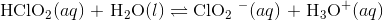According to the Brønsted-Lowry definition of acids and bases, which species in the reaction are acids?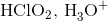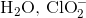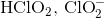There are no acids in the reaction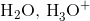In the forward reaction,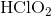is a proton donor. In the backward reaction,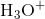is the proton donor.
The acids in this reaction areand.